# Training Neural Networks Explained SimplyTraining. Source: Uri Almog Instagram

In this post we will explore the mechanism of neural network training, but I’ll do my best to avoid rigorous mathematical discussions and keep it intuitive.

Consider the following task: you receive an image, and want an algorithm that returns (predicts) the correct number of people in the image.

We start by assuming that there is, indeed, some mathematical function out there that relates the collection of all possible images, with the collection of integer values describing the number of people in each image. We accept the fact that we will never know the actual function, but we hope to learn a model with finite complexity that approximates this function well enough.

Let’s assume that you’ve constructed some kind of neural network to perform this task. For the sake of this discussion, it’s not really important how many layers are there in the network or the nature of mathematical manipulations carried out in each layer. What is important, however, is that in the end there is one output neuron that predicts a (non-negative, hopefully integer) value.

The mathematical operation of the network can be expressed as a function:

f(x, w) = y

where x is the input image (we can think of it as a vector containing all the pixel values), y is the network’s prediction, and w is a vector containing all the internal parameters of the function (e.g. in f(x, w) = a + bx + exp(c*x) = y, the values of a, b and c are the parameters of the function).

As we saw in the post on perceptrons, during training we want some kind of mechanism that:

1. Evaluates the network’s prediction on a given input,
2. Compares it to the desired answer (the ground truth),
3. Produces a feedback that corresponds to the magnitude of the error,
4. And finally, modifies the network parameters in a way that improves its prediction (decreases the error magnitude).

And thanks to some clever minds — we have such mechanism. In order to understand it we need to cover two topics:

1. Loss function
2. Backpropagation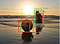Errors in object localization leading to loss during training. Source: Uri Almog Instagram

# Loss Function

Simply put, the loss function is the error magnitude. In more details, a good loss function should be a metric i.e. it defines a distance between points in the space of prediction values. — You can read more about distance functions here.

We would like to use a loss function that returns a small value when the network’s prediction is close to the ground truth, and large when it is far from the ground truth.

The aim of the loss function is to tell our training mechanism how big the error is. If there are 6 people in the image and our network predicted only 1, it’s a bigger error than if the network predicted 5. So it would be a logical choice to use as a loss function

L(y, y’) = abs(y-y’),

where y an y’ are the network prediction and the ground truth, respectively.

A more common choice is:

L(y, y’) = (y-y’)²

This function is preferred because of two important properties:

1. Its emphasis on large errors is larger than just linear,
2. It is smooth everywhere, even at y=y’. We will soon understand why this is important.

The value we are actually interested in is not the loss itself. What we want our training mechanism to calculate is the loss derivative. ‘Derivative with respect to what?’, you’re probably asking. And here comes the heart of the matter: contrary to what many machine learning beginners might think, we don’t derive the loss with respect to the input value x. For us, the input is a given, independent value. Instead, we derive the loss with respect to the network parameters w.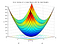Loss function over two parameters. Source: Wikipedia

Why?

Because the loss derivative, or gradient with respect to network parameters, dL/dw, tells us how much the loss changes (on the given input x), if we change the network parameters slightly. If we imagine the loss function as a landscape with hills and valleys on the parameter space (it’s easy to do it with just two parameters, but remember that in practice there are usually millions of parameters), then the loss gradient is a vector that points uphill. If we want to decrease the error we should move (i.e. slightly change the parameters) in the negative gradient direction — downhill.

Now it is clear why we prefer derivable functions. Using functions with non smooth properties can cause issues if not done carefully.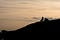Let’s go downhill! Source: Uri Almog Photography

How can we derive an unknown function?

We will never know the real underlying function for the task. What we have is a model function learning to approximate that underlying function. And that model function can be derived numerically by slightly changing each parameter separately and calculating the change to the loss.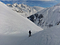Looking for a local minimum. Source: Uri Almog Instagram

# Backpropagation

Backpropagation, or back propagation, is a clever way to perform the numeric derivation process. We will not go into detail, but it’s basically picking each of the network parameters at a time (starting from the output layers and moving backward), and calculating the loss derivative according to the chain rule. You can read more on backpropagation here.

Deep learning frameworks have built-in methods for backpropagation, so luckily we don’t need to implement it ourselves each time we want to build and train a network.

Once we have the Loss gradient, we update the network parameters:

w_new = w — lr * grad(L)

where w_new is the updated value of the parameters vector, grad(L) is the loss gradient with respect to the parameters, and lr is the learning rate: a coefficient that controls the step size of the update. It’s a hyperparameter set by the user and usually decreases over the training stages according to a preset scheduling.

By updating the parameters, we’ve completed a single training step, and are now ready to repeat the process with another data point.

Each full cycle through the training set is called an epoch. In a typical training process there can be hundreds or thousands of epochs, depending on the case.

# When to stop training?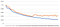Training loss (blue) and validation loss (red) over training steps. After step 17 the training is not effective.

We use two indicators to understand whether the network is training effectively:

1. Monitoring the loss: When a network trains effectively, we expect to see a decrease of the loss over time. If the loss doesn’t decrease, it may mean that the network has converged to a deep minimum point, or that the learning rate is too high and the network keeps missing the minima in the loss landscape.
2. Running a validation test: At fixed periods, run the network on inference mode (using the network for prediction without updating parameters) on a validation set — a collection of images that is not a part of the training set. We expect the loss to decrease on that set as well. If the loss decreases on the trainings set but not on the validation set, it means that the network is starting to overfit — it is learning the attributes of the training examples but isn’t learning to generalize and its performance on unseen data will eventually degrade. This occurs when the training set is too small or the network has too many parameters.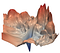Loss landscape of an actual neural network (ResNet56 without skip connections), projected on 2 parameters. Source: Visualizing the Loss Landscape of Neural Nets

# A few more words about training

This post is meant to give the basic concepts of neural network training. Beyond the basics, things get complicated very quickly.

1. Training a network belongs to a section in math and engineering called optimization. You probably encountered optimization problems in math class at school where you were required to find the shape of a field with the largest area etc. Analytically these problems require setting the derivative of some function to zero and solving it for the required properties (e.g. field length, width etc.). In real life problems we can’t always calculate the derivative but we can approximate it. That’s what we do in network training: we define a loss function which, for a perfect model, would have its parameters at a minimum point. The goal of the training process is to end up at a point in parameter space which is at a deep enough local minimum of the loss function.
2. Usually neural networks predict more than a single value: detection networks predict thousands of values per image, corresponding to object bounding box coordinates, confidence levels, object classes. Image enhancement networks literally predict whole images, etc. Generally the loss will have multiple dependencies on the different output features: e.g. an object detection network will have a loss for missing objects, but also for objects that were not localized correctly, and for objects that were not classified correctly. Choosing a good loss function is more than just math and engineering, it’s art. Two ML engineers working on the same network architecture and the same task can design different loss functions. Through the loss function you sculpture the network to learn features in a certain way. Some loss functions, may not be good enough, in the sense that it’s hard to converge to their local minima. Some loss functions contain complex relations between different output nodes of the network, or loss coefficients that change at different phases of the training (e.g. Yolo).
3. Usually training steps are performed on more than one data point at a time. The average loss is calculated over a batch of inputs, resulting in a smoother loss landscape (and better utilizing parallel computation techniques for higher speed).
4. As can be seen in the loss landscape image above, finding the deepest minimum is not as simple as letting a marble roll into a pit. A typical loss landscape has many crevasses, canyons and saddle points that will divert us from the root to the deepest point. Furthermore, we don’t expect to find The global minimum even in a successful training. We are satisfied with one of the many local minima that give good results on the data.
5. There are many heuristics on how to change the learning rate during training. Too big a step will prevent convergence, while too small a step will make the training very long and may get stuck in less than optimal minima. A common method is exponential decay (multiplying lr by a fixed factor < 1 at fixed training intervals). Another method changes lr in a cosine manner, either just half cycle during the training, or periodically. See some examples here. Beside learning rate, there are other tricks to control the dynamics of the convergence, such as momentum (using a weighted average of the current and previous gradients).
6. In order to help the network generalize (and also increase the effective size of the training set), we usually add random augmentations to the training images — e.g. — changing brightness, flipping the image horizontally, adding noise — as long as the resulting image is a valid input for the task. This method is very helpful when your training data is insufficient or the images are highly correlated (as is the case when you use frames taken from a video clip).

A machine learning engineer writing about computer vision. Loves to trek, scubadive, visual arts and combinations thereof. https://www.linkedin.com/in/urialmog/

## More from Uri Almog

A machine learning engineer writing about computer vision. Loves to trek, scubadive, visual arts and combinations thereof. https://www.linkedin.com/in/urialmog/